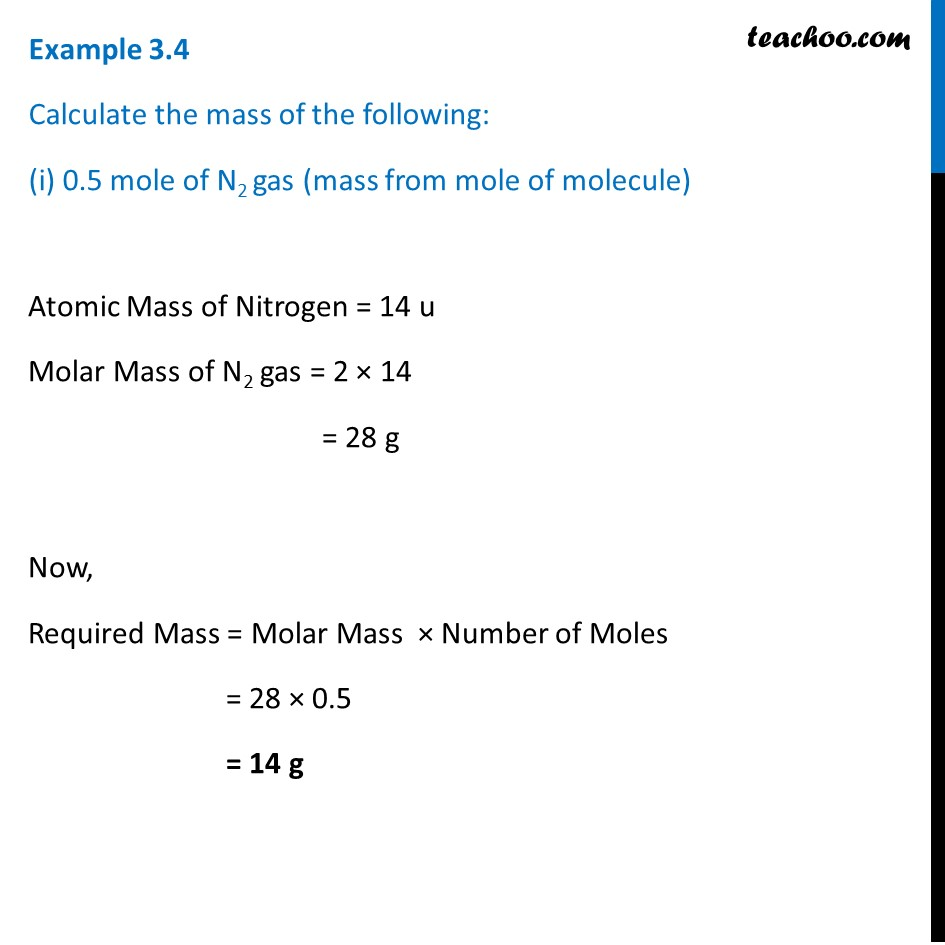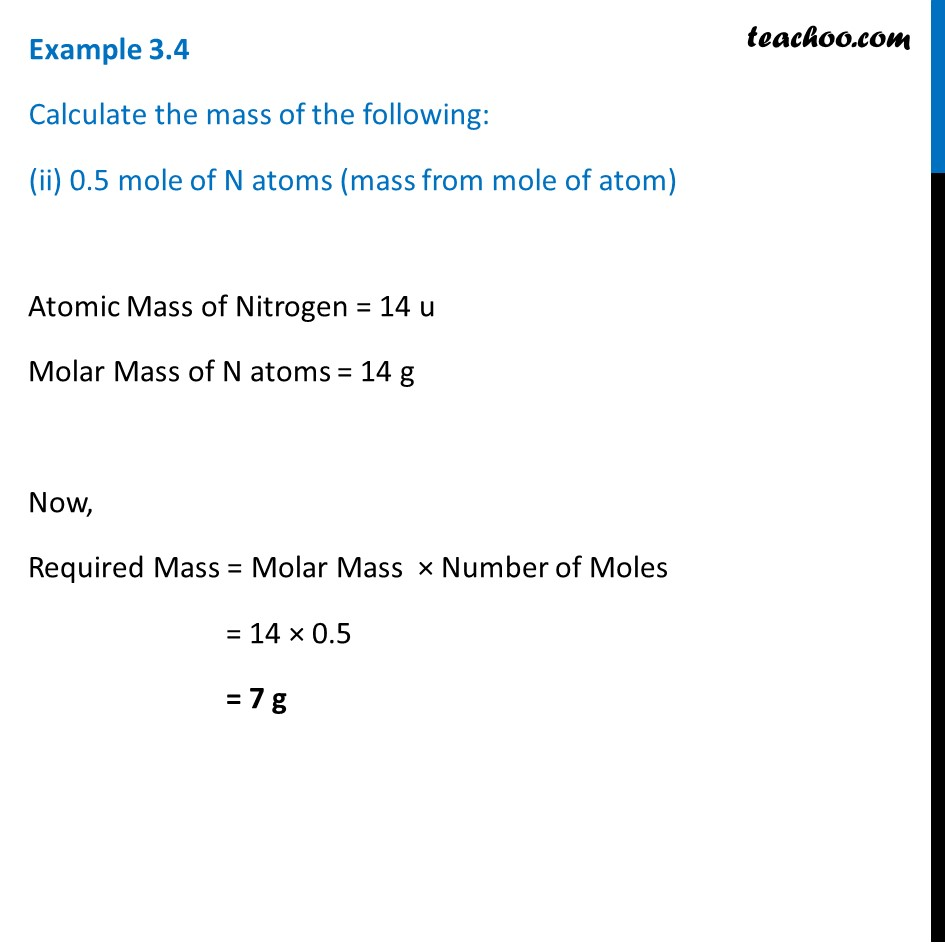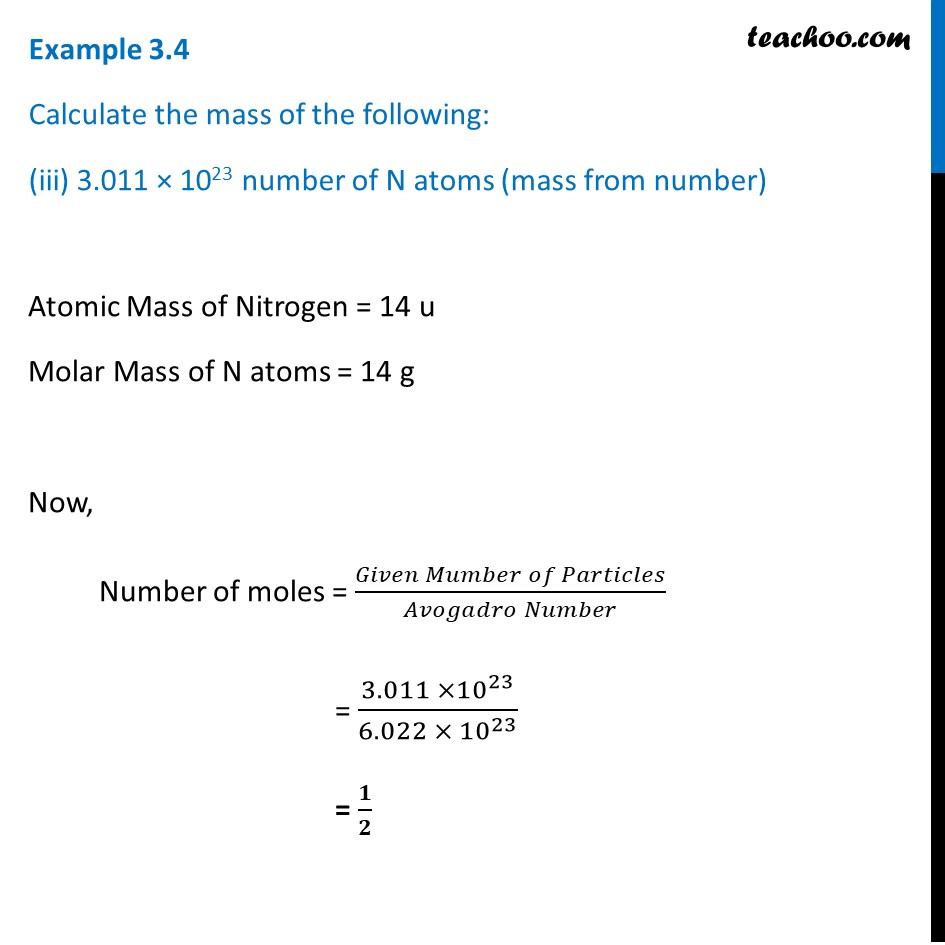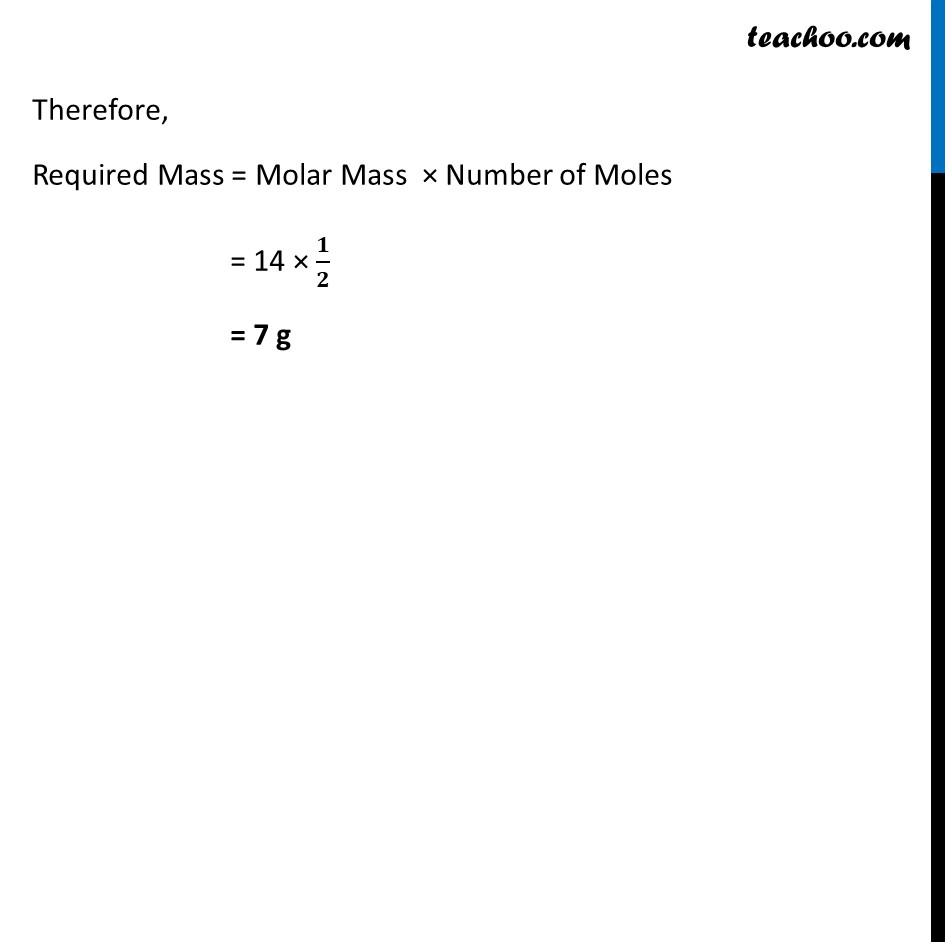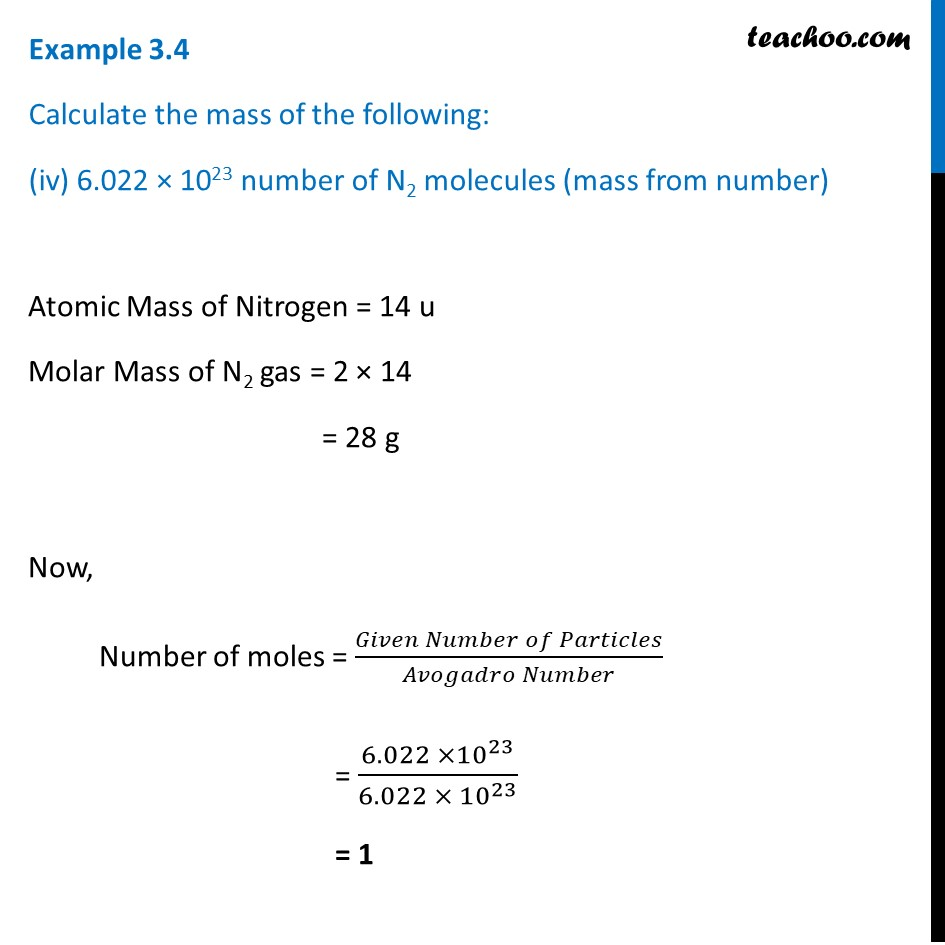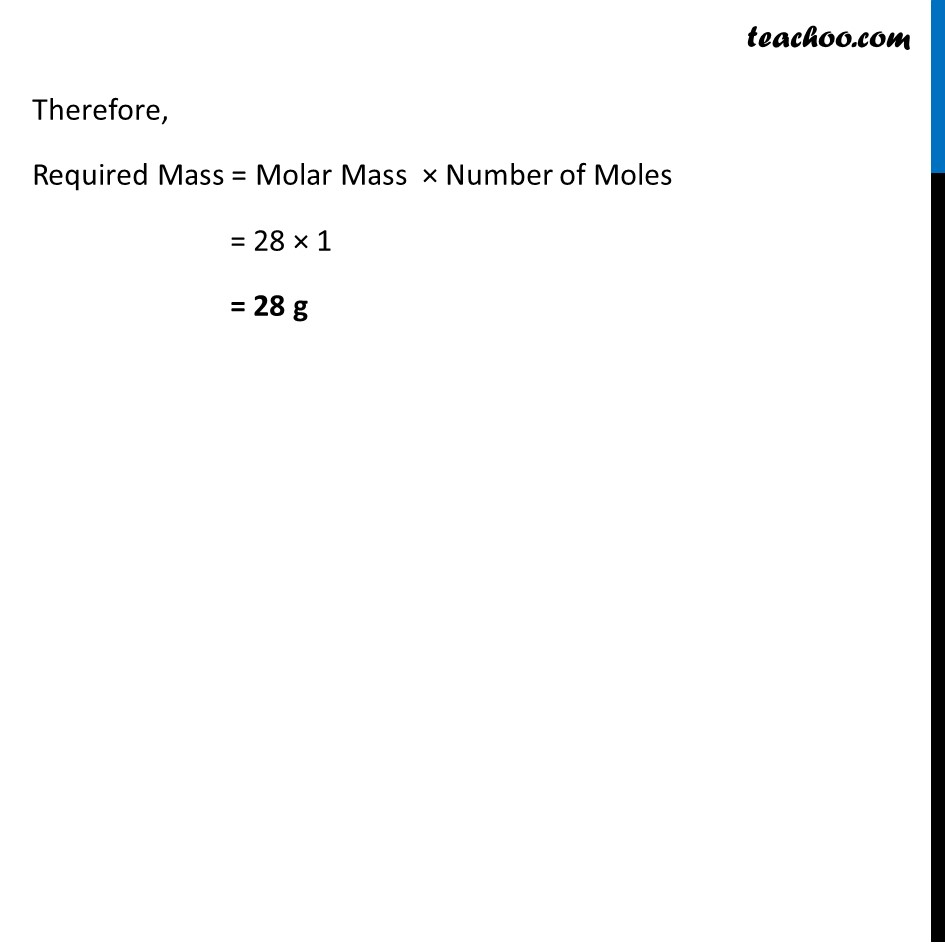1. Class 9
2. Chapter 3 Class 9 - Atoms And Molecules (Term 2)
3. Examples from NCERT Book

Transcript

Example 3.4 Calculate the mass of the following: (i) 0.5 mole of N2 gas (mass from mole of molecule) Atomic Mass of Nitrogen = 14 u Molar Mass of N2 gas = 2 × 14 = 28 g Now, Required Mass = Molar Mass × Number of Moles = 28 × 0.5 = 14 g Example 3.4 Calculate the mass of the following: (ii) 0.5 mole of N atoms (mass from mole of atom) Atomic Mass of Nitrogen = 14 u Molar Mass of N atoms = 14 g Now, Required Mass = Molar Mass × Number of Moles = 14 × 0.5 = 7 g Example 3.4 Calculate the mass of the following: (iii) 3.011 × 1023 number of N atoms (mass from number) Atomic Mass of Nitrogen = 14 u Molar Mass of N atoms = 14 g Now, Number of moles = (𝐺𝑖𝑣𝑒𝑛 𝑀𝑢𝑚𝑏𝑒𝑟 𝑜𝑓 𝑃𝑎𝑟𝑡𝑖𝑐𝑙𝑒𝑠)/(𝐴𝑣𝑜𝑔𝑎𝑑𝑟𝑜 𝑁𝑢𝑚𝑏𝑒𝑟) = (3.011 ×10^23)/(6.022 × 10^23 ) = 𝟏/𝟐 Therefore, Required Mass = Molar Mass × Number of Moles = 14 × 𝟏/𝟐 = 7 g Example 3.4 Calculate the mass of the following: (iv) 6.022 × 1023 number of N2 molecules (mass from number) Atomic Mass of Nitrogen = 14 u Molar Mass of N2 gas = 2 × 14 = 28 g Now, Number of moles = (𝐺𝑖𝑣𝑒𝑛 𝑁𝑢𝑚𝑏𝑒𝑟 𝑜𝑓 𝑃𝑎𝑟𝑡𝑖𝑐𝑙𝑒𝑠)/(𝐴𝑣𝑜𝑔𝑎𝑑𝑟𝑜 𝑁𝑢𝑚𝑏𝑒𝑟) = (6.022 ×10^23)/(6.022 × 10^23 ) = 1 Therefore, Required Mass = Molar Mass × Number of Moles = 28 × 1 = 28 g

Examples from NCERT Book

Class 9
Chapter 3 Class 9 - Atoms And Molecules (Term 2)